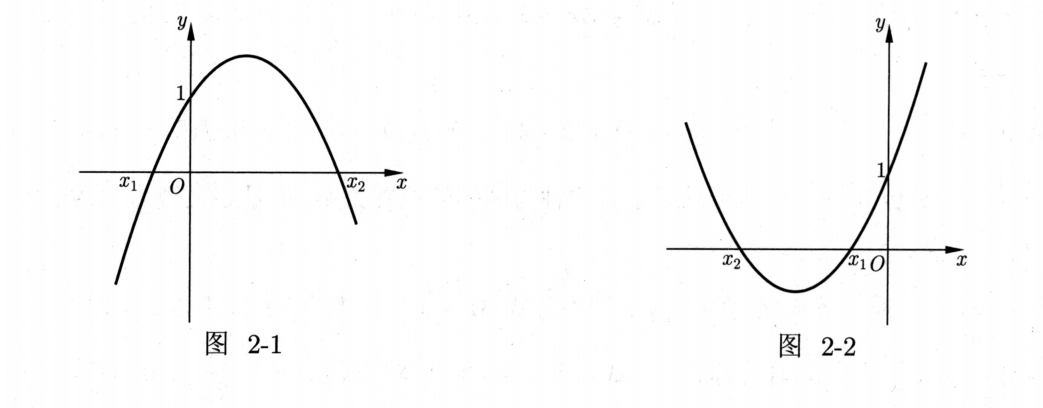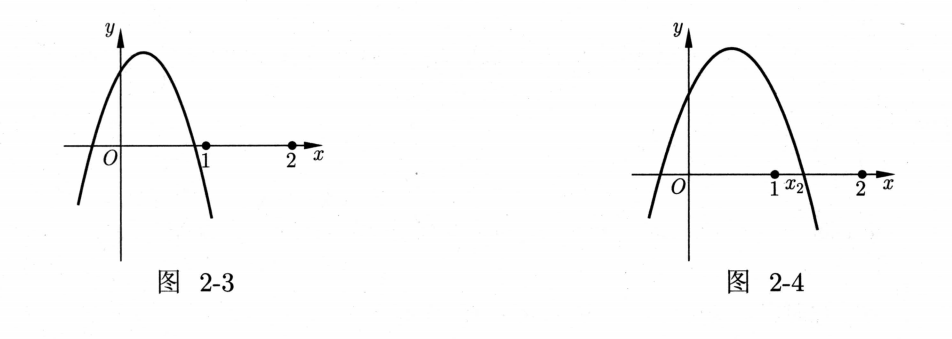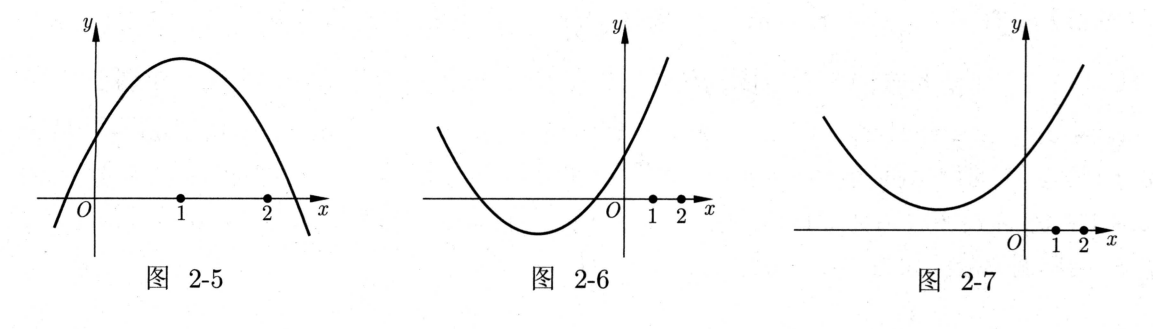• ## 导数的应用

千次阅读 2021-06-16 10:43:17
导数的应用        导数之所以重要，是因为利用导数能够很简洁地刻画函数的单调性、极值和最值等。 单调性        贯穿本小节的是函数 f(x)...
导数导数的应用单调性【知识点 1】【方法总结 1】【方法总结 2】【方法总结 3】极值与最值【知识点 2】【知识点 3】【知识点 4】 求函数 $f(x)$ 在 $[a, b]$ 上的最大值与最小值的步骤变式参考答案练习题练习题答案
导数的应用
导数之所以重要，是因为利用导数能够很简洁地刻画函数的单调性、极值和最值等。
单调性
贯穿本小节的是函数 $f(x)$ 的单调性与其导数 $f^{\prime}(x)$ 的关系。
【知识点 1】

若函数 $f(x)$ 在 $(a, b)$ 上可导，则
（1）$f(x)$ 在 $(a, b)$ 上单调递增 $\Leftrightarrow f^{\prime}(x) \geqslant 0$ 在 $(a, b)$ 上恒成立;
（2）$f(x)$ 在 $(a, b)$ 上单调递减 $\Leftrightarrow f^{\prime}(x) \leqslant 0$ 在 $(a, b)$ 上恒成立。

通过导数判断单调性，实质就是解关于导函数的不等式，并需要注意函数的定义域，这个细节看似平常却是决定成败的关键因素。

【例 2.8】 （2019 全国 Ⅱ 理 20）已知函数 $f(x)=\ln x-\frac{x+1}{x-1}$，讨论 $f(x)$ 的单调性。

【解析】 函数问题定义域先行，根据题意可知函数定义域为 $(0,1) \cup(1,+\infty)$，对函数求导可得 $f^{\prime}(x)=\frac{1}{x}+\frac{2}{(x-1)^{2}}=\frac{x^{2}+1}{x(x-1)^{2}}>0$，由此可知函数在 $(0,1)$，$(1,+\infty)$ 上单调递增。

【注】 若所求的函数单调区间不止一个的时候，不能用 $\cup$ 来连接。

【变式 1】 （2016 全国 Ⅱ 理 21）已知函数 $f(x)=\frac{x-2}{x+2} \mathrm{e}^{x}$，讨论 $f(x)$ 的单调性。

【变式 2】（2017 课标 Ⅱ 文 21）设函数 $f(x)=\left(1-x^{2}\right) \mathrm{e}^{x}$，讨论 $f(x)$ 的单调性。

不含参数的单调性问题中毕竟是少数，多数情形单调性问题都是围绕着参数来分类讨论，下面我们主要是围绕着含参的一元一次不等式和一元二次不等式来展开讨论。
当我们去解含参的一元一次不等式 $a x>b$ 时，不等式的解集由 $a$ 的符号决定。因此我们需要对 $a$ 分类讨论：
（1）当 $a>0$ 时，$x>\frac{b}{a}$；（2）当 $a<0$ 时，$x<\frac{b}{a}$；（3）当 $a=0$，$b<0$ 时，不等式的解集为 $\mathbb{R}$；（4）当 $a=0$，$b \geqslant 0$ 时，不等式无解。
【方法总结 1】

解一元一次不等式 $a x>b$，需要按 $a>0，a=0$ 和 $a<0$ 来分类讨论。

可能有读者想问了，这么简单的东西谁不会啊。

【例 2.9】（2009 北京理 18）已知函数 $f(x)=x \mathrm{e}^{k x}(k \neq 0)$，求函数 $f(x)$ 的单调区间。

【解析】 $f^{\prime}(x)=(k x+1) \mathrm{e}^{k x}$，其中 $\mathrm{e}^{k x}>0$ 恒成立。
（1）当 $k>0$ 时，因为 $f^{\prime}(x) \geqslant 0 \Leftrightarrow x \geqslant-\frac{1}{k}$，所以 $f(x)$ 在 $\left(-\frac{1}{k},+\infty\right)$ 上单调递增。同样地，$f^{\prime}(x) \leqslant 0 \Leftrightarrow x \leqslant-\frac{1}{k}$，因此 $f(x)$ 在 $\left(-\infty,-\frac{1}{k}\right)$ 上单调递减。
（2）当 $k<0$ 时，因为 $f^{\prime}(x) \geqslant 0 \Leftrightarrow x \leqslant-\frac{1}{k}$，所以 $f(x)$ 在 $\left(-\infty,-\frac{1}{k}\right]$ 上单调递增。同样地，$f^{\prime}(x) \leqslant 0 \Leftrightarrow x \geqslant-\frac{1}{k}$，因此 $f(x)$ 在 $\left[-\frac{1}{k},+\infty\right)$ 上单调递减。

不难看出，在例 2.9 中，自变量 $x$ 没有限制，也就是 $x \in \mathbb{R}$，因此我们没有必要去讨论它的解集。如果 $x$ 的范围不是 $\mathbb{R}$，我们肯定需要作更细致的讨论。
现在我们来看关于 $x$ 的不等式 $a x>b$，其中 $x \in[m, n]$。
显然，我们不仅要讨论 $a$ 的正负，还需要讨论其解集的端点 $\frac{b}{a}$ 是否落在区间 $[m, n]$ 内，看似很复杂，但是其思想很简单，我们总结如下:
【方法总结 2】

解一元一次不等式 $a x>b$，其中 $x \in[m, n]$，先按 $a>0$，$a=0$ 和 $a<0$ 来分 类讨论，然后再讨论 $\frac{b}{a}$ 是否落在区间 $[m, n]$ 内。

当然，一般不需要讨论这么多。

【例 2.10】（2016 山东文 20）设 $f(x)=x \ln x-a x^{2}+(2 a-1) x, a \in \mathbb{R}$。 令 $g(x)=f^{\prime}(x)$，求 $g(x)$ 的单调区间。

【解析】 函数的定义域为 $(0,+\infty)$。由题意可知 $g(x)=f^{\prime}(x)=\ln x-2 a x+2 a$，则 $g^{\prime}(x)= \frac{1}{x}-2 a=\frac{1-2 a x}{x}$ 。因此 $g(x)$ 的单调性实质上由一元一次函数 $y=1-2 a x$ 的符号决定。
（1）当 $a \leqslant 0$ 时，因为 $x>0$，所以 $1-2 a x>0$ 恒成立，从而 $g^{\prime}(x)>0$ 恒成立，函数 $g(x)$ 在 $(0,+\infty)$ 上单调递增。
（2）当 $a>0$ 时，因为 $g^{\prime}(x) \geqslant 0 \Leftrightarrow x \leqslant \frac{1}{2 a}$，所以 $g(x)$ 在 $\left(0, \frac{1}{2 a}\right]$ 上单调递增；又因为 $g^{\prime}(x) \leqslant 0 \Leftrightarrow x \geqslant \frac{1}{2 a}$，所以 $g(x)$ 在 $\left[\frac{1}{2 a},+\infty\right)$ 上单调递减。

【变式 3】（2015 新课标 Ⅱ 文 21）已知 $f(x)=\ln x+a(1-x)$，讨论 $f(x)$ 的单调性。

【变式 4】（2017 全国 Ⅲ 文 21）已知 $f(x)=\ln x+a x^{2}+(2 a+1) x$，讨论 $f(x)$ 的单调性。

一元一次不等式或者一次型函数不等式其实出现的频率并不是很高。往往都是涉及解二次不等式或者是二次型函数的不等式。对于一元二次不等式 $a x^{2}+b x+c>0$，不可能 $a$，$b$，$c$ 都是参数，一般只有一个。但是往往都有区间的限制，所以按部就班的分类讨论的次数也不会少。
在一次函数那里的套路只能将就用到一次函数那里，对于二次函数，我们得另辟罢径了，也就是接下来要讲的“临界条件”。
从字面意思理解就是从一种状态转变到另外一种状态时所要满足的条件。比如 $a x>b$，当 $a>0$ 和 $a<0$ 时，它的解是由 $\left(\frac{b}{a},+\infty\right)$ 转变到 $\left(-\infty, \frac{b}{a}\right)$，即解的方向从一个方向转变到另外一个方向，此时我们就说它的临界条件为 $a=0$。
那么，对于二次函数呢？情况要稍微复杂一点，为了方便读者理解，我们用以下的三个例子作解释。

【例 2.11】 解关于 $x$ 的不等式：
（Ⅰ）$3 x^{2}+a x+1>0$； （Ⅱ）$a x^{2}+3 x+1>0$； （Ⅲ）$a x^{2}+3 x+1>0, x \in[1,2]$。

【解析】 （Ⅰ）因为二次函数 $f(x)=3 x^{2}+a x+1$ 的二次项系数确定，所以只需考虑判别式 $\Delta=a^{2}-12$ 的符号，显然其临界条件为 $\Delta=0$，解得 $a=2 \sqrt{3}$ 或 $a=-2 \sqrt{3}$。
（1）当 $a<-2 \sqrt{3}$ 时，此时 $\Delta=a^{2}-12>0$，因此 $f(x)=0$ 有两个不等的实根，且两根分别为 $x_{1}=\frac{-a-\sqrt{a^{2}-12}}{6}$，$x_{2}=\frac{-a+\sqrt{a^{2}-12}}{6}$，显然 $x_{1}。因为 $f(x)$ 的图像开口向上，所以 $f(x)>0$ 的解集为 $\left\{x \mid x<\frac{-a-\sqrt{a^{2}-12}}{6}\right. 或 \left.x>\frac{-a+\sqrt{a^{2}-12}}{6}\right\}$。
（2）当 $a=-2 \sqrt{3}$ 时，此时不等式为 $3 x^{2}-2 \sqrt{3} x+1>0$，解得 $\left\{x \mid x \in \mathbb{R}, x \neq \frac{\sqrt{3}}{3}\right\}$ 。
（3）当 $-2 \sqrt{3} 时，此时 $\Delta=a^{2}-12<0$，因此 $f(x)=0$ 没有实数根。又因为它的开口向上，所以不等式的解集为 $\mathbb{R}$ 。
（4）当 $a=2 \sqrt{3}$ 时，此时不等式为 $3 x^{2}+2 \sqrt{3} x+1>0$，解得 $\left\{x \mid x \in \mathbb{R}, x \neq-\frac{\sqrt{3}}{3}\right\}$ 。
（5）当 $a>2 \sqrt{3}$ 时，此时 $\Delta=a^{2}-12>0$，因此 $f(x)=0$ 有两个不等的实根，且两根分别为 $x_{1}=\frac{-a-\sqrt{a^{2}-12}}{6}，x_{2}=\frac{-a+\sqrt{a^{2}-12}}{6}$，显然 $x_{1}。 因为 $f(x)$ 的图像开口向上，所以 $f(x)>0$ 的解集为 $\left\{x \mid x<\frac{-a-\sqrt{a^{2}-12}}{6}\right. 或 \left.x>\frac{-a+\sqrt{a^{2}-12}}{6}\right\}$。

【注】 显然，这里 $a>2 \sqrt{3}$ 和 $a<-2 \sqrt{3}$ 是可以合并在一起的，因为此时它们都满足 $\Delta>0 $；$a=2 \sqrt{3}$ 和 $a=-2 \sqrt{3}$ 也是可以合并在一起的，因为此时 $\Delta=0$。这里分开写是为了让读者思路清晰，在后面的变式训练中，我们将它们合并在一起，希望不会给读者带来任何疑惑。
（Ⅱ）因为函数 $f(x)=a x^{2}+3 x+1$ 的开口方向不确定，因此我们要同时考虑其开口方向与判别式，故其临界条件为 $\begin{cases} a=0 \\ \Delta=9-4 a=0\end{cases}$，即 $\begin{cases} a=0 \\ a=\frac{9}{4}\end{cases}$。
（1）当 $a<0$ 时，此时开口向下且 $\Delta=9-4 a>0$，因此 $f(x)=0$ 的两根为 $x_{1}=\frac{-3+\sqrt{9-4 a}}{2 a}$，$x_{2}=\frac{-3-\sqrt{9-4 a}}{2 a}$，且 $x_{1}。由图 2-1 可知，$f(x)>0$ 的解集为 $\left\{x \mid \frac{-3+\sqrt{9-4 a}}{2 a}。
（2）当 $a=0$ 时，原不等式化简为一元一次不等式 $3 x+1>0$，解得 $\left\{x \mid x>-\frac{1}{3}\right\}$。
（3）当 $0 时，此时开口向上且 $\Delta>0$，因此 $f(x)=0$ 的两根为 $x_{1}=\frac{-3+\sqrt{9-4 a}}{2 a}$，$x_{2}=\frac{-3-\sqrt{9-4 a}}{2 a}$，且 $x_{1}>x_{2}(a>0)$。由图 2-2 可知，$f(x)>0$ 的解集为 $\left\{x \mid x<\frac{-3-\sqrt{9-4 a}}{2 a}\right. 或 \left.x>\frac{-3+\sqrt{9-4 a}}{2 a}\right\}$。
（4）当 $a=\frac{9}{4}$ 时，原不等式化简为 $\frac{9}{4} x^{2}+3 x+1>0$，解得 $\left\{x \mid x \in \mathbb{R}，x \neq-\frac{2}{3}\right\}$。
（5）当 $a>\frac{9}{4}$ 时，此时开口向上，$\Delta=9-4 a<0$，故解集为 $\mathbb{R}$ 。（Ⅲ）因为函数 $f(x)=a x^{2}+3 x+1$ 的系数不确定，所以我们首先要考虑其开口方向与判别式，即 $\begin{cases} a=0 \\ \Delta=9-4 a=0\end{cases}$，还要考虑其解集是否与区间 $[1,2]$ 相交，此时的临界条件为 $\begin{cases} f(1)=0 \\ f(2)=0\end{cases}$ 。综合看来其临界条件为 $\begin{cases} a=0 \\ \Delta=9-4 a=0 \\ f(1)=0 \\ f(2)=0\end{cases}$，解得 $\begin{cases} a=0 \\ a=\frac{9}{4} \\ a=-4 \\ a=-\frac{7}{4}\end{cases}$。
（1）当 $a<-4$ 时，此时开口向下，$\Delta=9-4 a>0$，对称轴 $x=-\frac{3}{2 a}<1，f(1)=a+4<0$，$f(2)=4 a+7<0$，由此可以确定 $f(x)$ 的图像，由图 2-3 可知，$f(x)>0$ 的解集为空集。
（2）当 $a=-4$ 时，此时不等式为 $-4 x^{2}+3 x+1>0$，解得 $-\frac{1}{4}，与区间 $[1,2]$ 无交集，故 $f(x)>0$ 的解集为空集。
（3）当 $-4 时，此时开口向下，$\Delta=9-4 a>0$，对称轴 $x=-\frac{3}{2 a}<1，f(1)=$ $a+4>0$ 且 $f(2)<0$，由此可以确定 $f(x)$ 的图像，如图 $2-4$ 所示，我们能求出图像中 $x_{2}$ 的值，因为 $\Delta=9-4 a>0$，所以 $f(x)=0$ 有两根且分别为 $\frac{-3+\sqrt{9-4 a}}{2 a}$ 和 $\frac{-3-\sqrt{9-4 a}}{2 a}$ 。又因为此时 $a<0$，所以 $\frac{-3+\sqrt{9-4 a}}{2 a}<\frac{-3-\sqrt{9-4 a}}{2 a}$，故 $x_{2}=\frac{-3-\sqrt{9-4 a}}{2 a}$ 。由图 2-4 可知，$f(x)>0$ 的解集为
$\left\{x \mid 1 \leqslant x<\frac{-3-\sqrt{9-4 a}}{2 a}\right\}$。（4）当 $a=-\frac{7}{4}$，此时不等式为 $-\frac{7}{4} x^{2}+3 x+1>0$，解得 $-\frac{2}{7}，与区间 $[1,2]$ 的交集为 $\{x \mid 1 \leqslant x<2\}$。
（5）当 $-\frac{7}{4} 时，此时开口向下，$\Delta=9-4 a>0$，$f(1)=a+4>0$，$f(2)=4 a+7>0$，对称轴 $x=-\frac{3}{2 a}>\frac{6}{7}$，如图 2-5 所示，虽然无法确定对称轴与区间的位置关系，但是定义域为 $[1,2]$，故 $f(x)>0$ 的解集为 $\{x \mid 1 \leqslant x \leqslant 2\}$。
（6）当 $a=0$ 时，原不等式化简为一元一次不等式 $3 x+1>0$，解集为 $\{x \mid 1 \leqslant x \leqslant 2\}$。
（7）当 $0 时，此时开口向上，$\Delta=9-4 a>0$，对称轴 $x=-\frac{3}{2 a}<0，f(1)=a+4>0$，$f(2)=4 a+7>0$，由此可以确定 $f(x)$ 的图像，如图 2-6 所示，故 $f(x)>0$ 的解集为 $\{x \mid 1 \leqslant x \leqslant 2\}$。
（8）当 $a=\frac{9}{4}$，此时不等式为 $\frac{9}{4} x^{2}+3 x+1>0$，解得 $x \in\left(-\infty,-\frac{2}{3}\right) \cup\left(-\frac{2}{3},+\infty\right)$，与区间 $[1,2]$ 的交集为 $\{x \mid 1 \leqslant x \leqslant 2\}$。
（9）当 $a>\frac{9}{4}$ 时，此时开口向上，$\Delta=9-4 a<0$，对称轴 $x=-\frac{3}{2 a}<1$，$f(1)=a+4>0$，$f(2)=4 a+7>0$，由此可以确定 $f(x)$ 的图像，如图 2-7 所示，故 $f(x)>0$ 的解集为 $\{x \mid 1 \leqslant x \leqslant 2\}$。显然上面的临界条件为我们的分类讨论提供了一个切入点，即如何将参数分类，这是掌握分类讨论的第一步，也是最重要的一步。我们先将上述做法总结如下：
【方法总结 3】

（1）二次项系数不含参数且自变量没有限制时，临界条件是 $\Delta=0$；
（2）二次项系数含有参数且自变量没有限制时，临界条件是二次项系数为零与 $\Delta=0$ 联立；
（3）二次项系数含参数且自变量有限制时，临界条件是二次项系数为零，$\Delta=0$ 与区间端点处的函数值为零同时联立。

我们刚刚说过，通过临界条件得到参数只是成功的第一步。我们还需要读者在接下来的具体讨论中有一个清晰的思路，完成整个分类讨论的过程! 一个自然的问题就出现了：有没有一个标准，或者一条主线可以知道我们顺利地完成了分类讨论？庆幸的是，有，而且还是这个 “临界条件”！看我们下面的解释。

【注】 回顾刚才讲过的例 2.11。第（Ⅰ）问中，因为参数不在系数上且自变量没有限制，所以其临界条件就是其判别式 $\Delta=0$，通过临界条件我们确定了如何将参数分类！再注意看我们的解析，你就会发现分类讨论的主线是 $\Delta>0$，$\Delta=0$ 和 $\Delta<0$，即每一步都是去验证 $\Delta$ 的符号，因此分类讨论的主线就是临界条件！
再来看第 Ⅱ 问，因为参数出现在二次项的系数上，且变量没有限制，所以其临界条件是二次项系数为零与 $\Delta=0$，通过临界条件我们确定了如何将参数分类！再注意看我们的解析，你就会发现分类讨论的主线是同时确定开口方向和 $\Delta$ 的符号，因此分类讨论的主线也是临界条件!
最后来看第 Ⅲ 问，不仅参数出现在二次项系数上，而且变量有限制，因此其临界条件是二次项系数为零，$\Delta=0$ 与区间端点处的函数值为零同时联立。再注意看我们的解析，你就会发现分类讨论的主线是同时确定开口方向，$\Delta$ 的符号和函数在区间端点的值的符号，因此分类讨论的主线还是临界条件！细心的读者会说，不对，你还考虑了对称轴！对，我们确实考虑了对称轴，但是对称轴只是辅助我们作图而已！或许有读者被例 2.11 的第 Ⅲ 问给审住了，太复杂了！但请放心，更注重考查我们的思维缜密度而不是机械的、繁琐的分类讨论。一般而言，分类情形不会超过 4 种。
为了书写的规范性，我们下面将临界条件的获取放到分析中，解析中我们直接分类讨论。

【例 2.12】 （2014 广东文 21）已知 $f(x)=\frac{1}{3} x^{3}+x^{2}+a x+1(a \in \mathbb{R})$，求 $f(x)$ 的单调区间。

【分析】 判断 $f(x)$ 的单调性等价于判断 $f^{\prime}(x)=x^{2}+2 x+a$ 的符号。因为二次函数 $f^{\prime}(x)$ 的开 口方向确定，且自变量没有限制，所以其临界条件只有 $\Delta=4-4 a=0$，即 $a=1$，而且分类讨论的主线是分别验证 $\Delta$ 的符号。

【解析】 $f(x)=\frac{1}{3} x^{3}+x^{2}+a x+1$，求导 $f^{\prime}(x)=x^{2}+2 x+a$，$\Delta=4(1-a)$。
（1）当 $a \geqslant 1$ 时，此时 $\Delta \leqslant 0$，因此 $f^{\prime}(x) \geqslant 0$ 在 $\mathbb{R}$ 上恒成立，所以 $f(x)$ 在 $\mathbb{R}$ 上单调递增。
（2）当 $a<1$ 时，此时 $\Delta>0$，故 $f^{\prime}(x)=0$ 有两根 $x_{1}=-1-\sqrt{1-a}$，$x_{2}=-1+\sqrt{1-a}$ 且 $x_{1}，因此 $f^{\prime}(x) \geqslant 0$ 的解区间为 $(-\infty,-1-\sqrt{1-a}]$ 和 $[-1+\sqrt{1-a},+\infty)$，即 $f(x)$ 的单调递增区间为 $(-\infty,-1-\sqrt{1-a}]$ 和 $[-1+\sqrt{1-a},+\infty)$ 。同样地，$f^{\prime}(x) \leqslant 0$ 的解区间为 $[-1-\sqrt{1-a},-1+\sqrt{1-a}]$，即 $f(x)$ 的单调递减区间为 $[-1-\sqrt{1-a},-1+\sqrt{1-a}]$ 。

若二次函数能直接分解因式，则我们一定要先分解因式。虽然它不会影响我们去寻找临界条件，但是会给我们后面具体的分类讨论带来一些差异。庆幸的是，它带给我们的是方便，而不是麻烦。

【例 2.13】（2015 江苏 19）已知函数 $f(x)=x^{3}+a x^{2}+b$，$a, b \in \mathbb{R}$，讨论 $f(x)$ 的单调性。

【分析】 求导可得 $f^{\prime}(x)=3 x^{2}+2 a x$，因为参数不在二次项系数上，且变量没有限制，故临界条件是 $\Delta=0$，即 $a=0$。另外，我们是可以将其分解因式的，即 $f^{\prime}(x)=3 x\left(x+\frac{2}{3} a\right)$。

【解析】 由题意，$f^{\prime}(x)=3 x^{2}+2 a x=3 x\left(x+\frac{2}{3} a\right)$。
（1）当 $a<0$ 时，$f^{\prime}(x)=0$ 的两根 $x_{1}=0$，$x_{2}=-\frac{2}{3} a>0$，则 $f(x)$ 在 $(-\infty, 0)$ 和 $\left(-\frac{2}{3} a,+\infty\right)$ 单调递增，在 $\left[0,-\frac{2}{3} a\right]$ 上单调递减；
（2）当 $a=0$ 时，$f^{\prime}(x)=3 x^{2} \geqslant 0$ 恒成立，所以 $f(x)$ 在 $\mathbb{R}$ 上单调递增；
（3）当 $a>0$ 时，$f^{\prime}(x)=0$ 的两根 $x_{1}=0$，$x_{2}=-\frac{2}{3} a<0$，可知 $f(x)$ 在 $\left(-\infty,-\frac{2}{3} a\right)$ 和 $(0,+\infty)$ 上单调递增，在 $\left[-\frac{2}{3} a, 0\right]$ 上单调递减。

【注】 从例 2.13 的解析可以看出我们虽然通过 $\Delta=0$ 去得到临界条件，但是我们在具体的分类讨 论的过程中，并没有像例 $2.12$ 那样，去讨论 $\Delta$ 的符号！为什么呢，因为只要二次函数能分解因式，其判别式 $\Delta$ 就不可能小于零，所以我们可以直接求根！这就是能分解因式的好处。以上我们讲的几个例子中，自变量都没有限制。下面我们再来看看自变量有限制的情形：

【例 2.14】（2009 江苏 20）已知 $f(x)=3 x^{2}-2 a x+a^{2}, x \in(a,+\infty)$，求 $f(x) \geqslant 1$ 的解集。

【分析】 令 $g(x)=f(x)-1=3 x^{2}-2 a x+a^{2}-1$，由于 $g(x)$ 的开口方向确定，故我们的临界条件为 $\begin{cases} \Delta=12-8 a^{2}=0 \\ g(a)=2 a^{2}-1=0\end{cases}$，解得 $\begin{cases} a=-\frac{\sqrt{6}}{2} \text { 或 } a=\frac{\sqrt{6}}{2} \\ a=-\frac{\sqrt{2}}{2} \text { 或 } a=\frac{\sqrt{2}}{2}\end{cases}$，注意到 $a \leqslant-\frac{\sqrt{6}}{2}$ 或 $a \geqslant \frac{\sqrt{6}}{2}$ 时，我们得到的都是 $\Delta \leqslant 0$，因此我们直接将这两种情形合并。另外，分类讨论的主线是先判断 $\Delta$ 和 $g(a)$ 的符号，然后通过图像判断，不过我们最好判断一下对称轴的位置，它能更精细的确定函数的图像。

【解析】 令 $g(x)=f(x)-1=3 x^{2}-2 a x+a^{2}-1$，故 $f(x) \geqslant 1$ 等价于 $g(x) \geqslant 0$。
（1）当 $a \leqslant-\frac{\sqrt{6}}{2}$ 或 $a \geqslant \frac{\sqrt{6}}{2}$ 时，此时 $\Delta \leqslant 0$，因此 $g(x) \geqslant 0$ 恒成立，即 $g(x) \geqslant 0$ 的解为 $\mathbb{R}$，显然 $\mathbb{R}$ 与 $(a,+\infty)$ 的交集为 $(a,+\infty)$，因此 $g(x) \geqslant 0$ 的解区间为 $(a,+\infty)$。
（2）当 $-\frac{\sqrt{6}}{2} 时，此时 $\Delta>0$，对称轴 $x=\frac{a}{3}>a，g(a)=2 a^{2}-1 \geqslant 0$。因为 $\Delta>0$，所以 $g(x)=0$ 有两个实数根，分别为 $x_{1}=\frac{a-\sqrt{3-2 a^{2}}}{3}$ 和 $x_{2}=\frac{a+\sqrt{3-2 a^{2}}}{3}$，且 $x_{1}，可知 $g(x) \geqslant 0$ 的解区间为 $\left(a, \frac{a-\sqrt{3-2 a^{2}}}{3}\right] \cup\left[\frac{a+\sqrt{3-2 a^{2}}}{3},+\infty\right)$ 。
（3）当 $-\frac{\sqrt{2}}{2} 时，此时 $\Delta>0$，$g(a)<0$ 。因为 $\Delta>0$，所以 $g(x)=0$ 有两个实数根，分别为 $x_{1}=\frac{a-\sqrt{3-2 a^{2}}}{3}$ 和 $x_{2}=\frac{a+\sqrt{3-2 a^{2}}}{3}$，且 $x_{1}，可知 $g(x) \geqslant 0$ 的解区间为 $\left[\frac{a+\sqrt{3-2 a^{2}}}{3},+\infty\right)$。
（4）当 $\frac{\sqrt{2}}{2} \leqslant a<\frac{\sqrt{6}}{2}$ 时，此时 $\Delta>0$，对称轴 $x=\frac{a}{3}，$g(a)>0$，可知 $g(x) \geqslant 0$ 的解区间为 $(a,+\infty)$。

例 2.14 中明确给出了区间的限制。但很多时候，这个区间限制需要我们自己挖掘，其中最常见的就是其定义域，见下例:

【例 2.15】 （2018 全国 Ⅰ 理 21）已知函数 $f(x)=\frac{1}{x}-x+a \ln x$，讨论 $f(x)$ 的单调性。

【分析】 讨论函数 $f(x)$ 的单调性就是讨论其导数 $f^{\prime}(x)=\frac{-x^{2}+a x-1}{x^{2}}$ 的符号，即讨论 $g(x)=-x^{2}+a x-1$ 的符号。此时一定要注意，虽然题中没有明确的区间限制，但是函数 $f(x)$ 是有定义域限制的，显然函数 $f(x)$ 定义域为 $x \in(0,+\infty)$，因此问题转化为讨论函数 $g(x)$ 在区间 $(0,+\infty)$ 上的符号，不难看出其分类讨论的临界条件是 $\begin{cases} \Delta=0 \\ g(0)=0\end{cases}$，解得 $\begin{cases} a=2 \\ a=-2\end{cases}$。另外，分类讨论的主线是先判断 $\Delta$ 和 $g(0)$ 的符号，然后通过图像判断，不过我们最好判断下对称轴的位置，它能更精细地确定函数的图像。

【解析】 $f(x)=\frac{1}{x}-x+a \ln x$，求导得 $f^{\prime}(x)=\frac{-x^{2}+a x-1}{x^{2}}(x>0)$，令 $g(x)=-x^{2}+a x-1(x>0)$，开口向下，$\Delta=a^{2}-4$。
（1）当 $-2 \leqslant a \leqslant 2$ 时，此时 $\Delta=a^{2}-4 \leqslant 0$，注意到开口向下，所以 $f^{\prime}(x) \leqslant 0$ 恒成立，即 $f(x)$ 在 $(0,+\infty)$ 上单调递减。
（2）当 $a<-2$ 时，此时 $\Delta=a^{2}-4>0$，对称轴 $x=\frac{a}{2}<-1，g(0)<0$ 。由此可知 $g(x)<0$ 在 $(0,+\infty)$ 上恒成立，即 $f(x)$ 在 $(0,+\infty)$ 上单调递减。
（3）当 $a>2$ 时，此时 $\Delta>0$，对称轴 $x=\frac{a}{2}>1，g(0)<0$，显然我们需要求出 $g(x)=0$ 的两个根 $x_{1}$ 和 $x_{2}$，$g(x)=0$ 的两根分别为 $x_{1}=\frac{a-\sqrt{a^{2}-4}}{2}$ 和 $x_{2}=\frac{a+\sqrt{a^{2}-4}}{2}$，显然 $x_{1}，因此 $g(x) \geqslant 0$ 在 $\left[\frac{a-\sqrt{a^{2}-4}}{2}，\frac{a+\sqrt{a^{2}-4}}{2}\right]$ 上恒成立，即 $f(x)$ 在 $\left[\frac{a-\sqrt{a^{2}-4}}{2}, \frac{a+\sqrt{a^{2}-4}}{2}\right]$ 上单调递增；同理，$g(x)<0$ 在 $\left(0, \frac{a-\sqrt{a^{2}-4}}{2}\right)$ 和 $\left(\frac{a+\sqrt{a^{2}-4}}{2},+\infty\right)$ 上恒成立，故 $f(x)$ 在 $\left(0, \frac{a-\sqrt{a^{2}-4}}{2}\right)$ 和 $\left(\frac{a+\sqrt{a^{2}-4}}{2},+\infty\right)$ 上单调递减。

接下来的例 $2.16$ 虽说表面上并不具有二次型，但却是披着指数外衣的一元二次函数的形式。

【例 2.16】 （2017 全国 Ⅰ 文 21）函数 $f(x)=\mathrm{e}^{x}\left(\mathrm{e}^{x}-a\right)-a^{2} x$。讨论函数 $f(x)$ 的单调性。

【分析】 函数 $f(x)$ 的定义域为 $\mathbb{R}$，$f^{\prime}(x)=2\left(\mathrm{e}^{x}\right)^{2}-a \mathrm{e}^{x}-a^{2}=\left(2 \mathrm{e}^{x}+a\right)\left(\mathrm{e}^{x}-a\right)$，令 $t=\mathrm{e}^{x}$，则 $f^{\prime}(t)=(2 t+a)(t-a)(t>0)$，实质为一元二次函数形式，临界条件是 $\begin{cases} \Delta=0 \\ f^{\prime}(0)=0\end{cases} \Rightarrow a=0$。

【解析】 $f(x)=\mathrm{e}^{x}\left(\mathrm{e}^{x}-a\right)-a^{2} x$，定义域为 $x \in \mathbb{R}, f^{\prime}(x)=2\left(\mathrm{e}^{x}\right)^{2}-a \mathrm{e}^{x}-a^{2}=\left(2 \mathrm{e}^{x}+a\right)\left(\mathrm{e}^{x}-a\right)$ 。
（1）当 $a=0$ 时，$f^{\prime}(x)=2\left(\mathrm{e}^{x}\right)^{2}>0$ 恒成立，$f(x)$ 在 $\mathbb{R}$ 上单调递增。
（2）当 $a>0$ 时，因为 $2 \mathrm{e}^{x}+a>0$，所以 $f^{\prime}(x)=0$ 的根为 $x=\ln a$。当 $x<\ln a$ 时，$f^{\prime}(x)<0$，$f(x)$ 单调递减，当 $x>\ln a$ 时，$f^{\prime}(x)>0$，$f(x)$ 单调递增。
（3）当 $a<0$ 时，因为 $\mathrm{e}^{x}-a>0$，所以 $f^{\prime}(x)=0$ 的根为 $x=\ln \left(-\frac{a}{2}\right)$。当 $x<\ln \left(-\frac{a}{2}\right)$ 时，$f^{\prime}(x)<0$，$f(x)$ 单调递减，当 $x>\ln \left(-\frac{a}{2}\right)$ 时，$f^{\prime}(x)>0$，$f(x)$ 单调递增。
综上可知，当 $a=0$ 时，$f(x)$ 在 $\mathbb{R}$ 上单调递增；当 $a>0$ 时，$f(x)$ 在 $(\ln a,+\infty)$ 上单调递增，在 $(-\infty, \ln a)$ 上单调递减；当 $a<0$ 时，$f(x)$ 在 $\left(\ln \left(-\frac{a}{2}\right),+\infty\right)$ 上单调递增，在 $\left(-\infty, \ln \left(-\frac{a}{2}\right)\right)$ 上单调递减。

【变式 5】（2016 天津理 20）设函数 $f(x)=(x-1)^{3}-a x-b, x \in \mathbb{R}$，其中 $a, b \in \mathbb{R}$。求 $f(x)$ 的单调区间。

【变式 6】（2016 山东理 20）已知函数 $f(x)=a(x-\ln x)+\frac{2 x-1}{x^{2}}, a \in \mathbb{R}$。讨论函数 $f(x)$ 的单调性。

极值与最值
在上一节我们阐述了函数 $f(x)$ 的导函数 $f^{\prime}(x)>0$，函数 $f(x)$ 单调递增，$f^{\prime}(x)<0$，函数 $f(x)$ 单调递减，那么 $f^{\prime}(x)=0$ 又有什么特殊意义呢？
【知识点 2】

若函数 $f(x)$ 在 $x_{0}$ 处取得极值，则 $f^{\prime}\left(x_{0}\right)=0$。

【例 2.17】（2018 新课标 Ⅰ 文 21）已知函数 $f(x)=a \mathrm{e}^{x}-\ln x-1$，设 $x=2$ 是 $f(x)$ 的极值点，求 $a$。

【解析】 对函数 $f(x)$ 求导可得 $f^{\prime}(x)=a \mathrm{e}^{x}-\frac{1}{x}$，因为 $x=2$ 是 $f(x)$ 的极值点，所以 $f^{\prime}(2)=0$,
即 $a \mathrm{e}^{2}-\frac{1}{2}=0$，解得 $a=\frac{1}{2 \mathrm{e}^{2}}$。

在明确 $x_{0}$ 为函数 $f(x)$ 的极值点的前提下，必有 $f^{\prime}\left(x_{0}\right)=0$。若 $f^{\prime}\left(x_{0}\right)=0$，并不能说明 $x_{0}$ 为函数
$f(x)$ 的极值点，还得判断 $f^{\prime}(x)$ 在 $x_{0}$ 两侧是否异号，总结如下：
【知识点 3】

若函数 $f(x)$ 在 $x_{0}$ 处满足 $f^{\prime}(x)=0$，则
（1）若在 $x_{0}$ 的左侧 $f^{\prime}(x)>0$，右侧 $f^{\prime}(x)<0$，则 $f\left(x_{0}\right)$ 是极大值，$x_{0}$ 是 $f(x)$ 的极大值点；
（2）若在 $x_{0}$ 的左侧 $f^{\prime}(x)<0$，右侧 $f^{\prime}(x)>0$，则 $f\left(x_{0}\right)$ 是极小值，$x_{0}$ 是 $f(x)$ 的极小值点；
（3）若 $f^{\prime}(x)$ 在 $x_{0}$ 两侧不变号，则 $f\left(x_{0}\right)$ 不是极值，$x_{0}$ 不是 $f(x)$ 的极值点。

【注】 特别注意，极值点并不是点，而是横坐标。

【例 2.18】 设函数 $f(x)$ 的导函数 $f^{\prime}(x)=x^{3}-3 x+2$，则函数 $f(x)$ 的极值点为（       ）。
A. $1$
B. $-2$
C. $1$ 或 $-2$
D. $1$ 或 $2$

【解析】 因为 $f^{\prime}(x)=x^{3}-3 x+2=(x-1)^{2}(x+2)$，所以 $f^{\prime}(x)=0$ 的根为 $x=1$ 或 $x=-2$，于是函数 $f(x)$ 的极值点只可能是 $x=1$ 或 $x=-2$ 。
（1）当 $x<-2$ 时，$f^{\prime}(x)<0$; 当 $-2 时，$f^{\prime}(x)>0$，所以 $x=-2$ 是 $f(x)$ 的极值点。
（2）当 $-2 或 $x>1$ 时，$f^{\prime}(x)>0$，所以 $x=1$ 不是函数 $f(x)$ 的极值点。
综上可知，$x=-2$ 是函数 $f(x)$ 的极值点，故选 B。

【例 2.19】（2019 重庆五月调研理 21）已知 $a \in \mathbb{R}$，函数 $f(x)=x^{2}+2 a x+(4-a) \ln x$ 有两个不同的极值点，求 $a$ 的取值范围。

【解析】 求导可得 $f^{\prime}(x)=2 x+2 a+\frac{4-a}{x}=\frac{2 x^{2}+2 a x+4-a}{x}$。令 $g(x)=2 x^{2}+2 a x+4-a(x>0)$，$f(x)$ 有两个极值点等价于 $g(x)=2 x^{2}+2 a x+4-a=0$ 在 $(0,+\infty)$ 有两个不同的实数根，则 $\begin{cases} \Delta=4 a^{2}-8(4-a)>0 \\ -\frac{a}{2}>0 \\ g(0)>0 & \end{cases}$，解得 $a<-4$。故实数 $a$ 的取值范围是 $(-\infty,-4)$。

【例 2.20】（2017 全国 Ⅱ 理 11）若 $x=-2$ 是函数 $f(x)=\left(x^{2}+a x-1\right) \mathrm{e}^{x-1}$ 的极值点，则 $f(x)$ 的极小值为（       ）。
A. $-1$
B. $-2 \mathrm{e}^{-3}$
C. $5 \mathrm{e}^{-3}$
D. $1$

【解析】 对函数 $f(x)$ 求导可得 $f^{\prime}(x)=\left[x^{2}+(a+2) x+a-1\right] \mathrm{e}^{x-1}$，$x=-2$ 是函数 $f(x)$ 的极值点，所以 $f^{\prime}(-2)=0$，可得 $a=-1$，可得 $f(x)=\left(x^{2}-x-1\right) \mathrm{e}^{x-1}，f^{\prime}(x)=\left(x^{2}+x-2\right) \mathrm{e}^{x-1}$。令 $f^{\prime}(x)=0$，解得 $x=-2$ 或 $x=1$。
（1）当 $x<-2$ 时，$f^{\prime}(x)>0$，当 $-2 时，$f^{\prime}(x)<0$，所以 $x=-2$ 是函数 $f(x)$ 的极大值点；
（2）当 $x>1$ 时，$f^{\prime}(x)>0$，当 $-2 时，$f^{\prime}(x)<0$，所以 $x=1$ 是函数 $f(x)$ 的极小值点，则 $f(1)=-1$，故选 A。

【变式 7】 设 $a \in \mathbb{R}$，若函数 $f(x)=\mathrm{e}^{a x}+3 x(x \in \mathbb{R})$ 有大于零的极值点，则（       ）。
A. $a>-3$
B. $a<-3$
C. $a>-\frac{1}{3}$
D. $a<-\frac{1}{3}$

【变式 8】（2018 北京理 18）设函数 $f(x)=\left[a x^{2}-(4 a+1) x+4 a+3\right] \mathrm{e}^{x}$，若 $f(x)$ 在 $x=2$ 处取得极小值，求 $a$ 的取值范围。

【知识点 4】 求函数 $f(x)$ 在 $[a, b]$ 上的最大值与最小值的步骤

（1）先确定 $f(x)$ 在 $(a, b)$ 上的所有可能的极值点，即先求 $f^{\prime}(x)$ 的所有零点，不妨设为 $x_{1}, \cdots, x_{n}$
（2）$f(x)_{\max }=\max \left\{f(a), f\left(x_{1}\right), \cdots, f\left(x_{n}\right), f(b)\right\}$，$f(x)_{\min }=\min \left\{f(a), f\left(x_{1}\right), \cdots, f\left(x_{n}\right), f(b)\right\}$。

求最值的过程中可以不去验证 $f\left(x_{1}\right), \cdots, f\left(x_{n}\right)$ 是否为函数 $f(x)$ 的极值，只判断它们的大小即可。

【例 2.21】（2014 四川文 21）已知函数 $f(x)=\mathrm{e}^{x}-a x^{2}-b x-1$，若 $g(x)$ 是函数 $f(x)$ 的导函数，求函数 $g(x)$ 在区间 $[0,1]$ 上的最小值。

【解析】 对函数 $f(x)$ 求导可得 $f^{\prime}(x)=\mathrm{e}^{x}-2 a x-b$，则 $g(x)=\mathrm{e}^{x}-2 a x-b$，可得 $g^{\prime}(x)=\mathrm{e}^{x}-2 a$